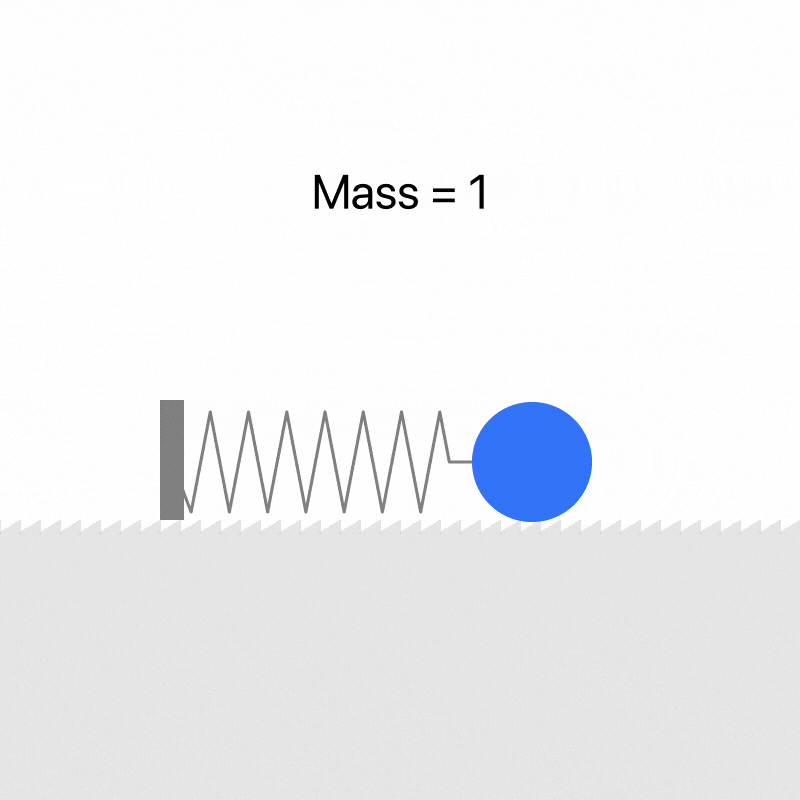#### 1. 在原型工具中设计弹性动效

1. Origami Studio2. Principle3. 其他△ ProtoPie Studio△ Flinto

#### 2. 在主流平台实现弹性动效

1. iOS

```UIView.animateWithDuration:usingSpringWithDamping:initialSpringVelocity

+ (void)animateWithDuration:(NSTimeInterval)duration
delay:(NSTimeInterval)delay
usingSpringWithDamping:(CGFloat)dampingRatio
initialSpringVelocity:(CGFloat)velocity
options:(UIViewAnimationOptions)options
animations:(void (^)(void))animations
completion:(void (^)(BOOL finished))completion;```

• dampingRatio：与弹性动效静止时的阻尼比。值为 1 时将得到平稳减速没有弹性的效果（即临界阻尼状态），值越接近 0 震荡（弹性）程度越大。velocity：弹性动效的初始速度。为了平滑地开始动效，请把这个值与之前附着的视图的速度匹配。

* 注：iOS 系统常用的临界阻尼效果，参数为：duration: 0.5, dampingRatio: 1

UIViewPropertyAnimator 的参数中提到：

The damping ratio for the spring is computed from the formula damping / (2 * sqrt (stiffness * mass)).

—— UISpringTimingParameters

2. Android

```findViewById(R.id.imageView).also { img ->
SpringAnimation(img, DynamicAnimation.TRANSLATION_X).apply {
…
spring.dampingRatio = SpringForce.DAMPING_RATIO_LOW_BOUNCY
spring.stiffness = SpringForce.STIFFNESS_LOW
setStartVelocity(velocity)
}
}```

SpringAnimation 提供了 3 个参数来定义弹性动效：dampingRatio、stiffness 和 setStartVelocity。

setStartVelocity：起始速度用于定义在动画开始时动画属性更改的速度。

dampingRatio：阻尼比用于描述弹簧震动逐渐衰减的状况。通过使用阻尼比，您可以定义震动从一次弹跳到下一次弹跳所衰减的速度有多快。以下列出了可使弹簧弹力衰减的四种不同方式：

• 当阻尼比大于 1 时，会出现过阻尼现象。它会使对象快速地返回到静止位置。
• 当阻尼比等于 1 时，会出现临界阻尼现象。这会使对象在最短时间内返回到静止位置。
• 当阻尼比小于 1 时，会出现欠阻尼现象。这会使对象多次经过并越过静止位置，然后逐渐到达静止位置。
• 当阻尼比等于零时，便会出现无阻尼现象。这会使对象永远震动下去。

stiffness：刚度定义了用于衡量弹簧强度的弹簧常量。不在静止位置的坚硬弹簧可对所连接的对象施加更大的力。

3. Web

`easing: 'spring(mass, stiffness, damping, velocity)'`

#### 3. 从设计到实现的差异

1. 设计工具2. 开发平台3. 差异

#### 4. 弹性系统原理

1. 什么是弹性系统

2. 质量（Mass）3. 阻尼（Damping）

4. 阻尼比（Damping Ratio）

• 无阻尼：阻尼比 -> 0，系统处于永远震荡的状态；
• 欠阻尼：阻尼比 < 1，对象进行指数递减的震荡运动；
• 过阻尼：阻尼比 > 1，对象进行无震荡的减速运动；
• 临界阻尼：阻尼比 = 1，对象以最短时间结束运动。5. 刚度（Stiffness）6. 摩擦力（Friction）

7. 张力（Tension）

#### 结论与成果

```damping = friction
stiffness = tension
[default]mass = 1
dampingRatio = damping / (2 * sqrt (mass * stiffness))
[default]velocity = 0```

1. 应用

2. Origami

• Bounciness = 5
• Speed = 10

• Friction = 27.0487
• Tension = 299.61884

• damping = 27.05
• stiffness = 299.62
• mass = 1
• velocity = 0
• dampingRatio = 0.78

For Android

```SpringAnimation(object, DynamicAnimation.[property]).apply {
…
spring.dampingRatio = 0.78f
spring.stiffness = 299.62f
setStartVelocity(0f)
}
```

For iOS

```let spring = CASpringAnimation(keyPath:[property])
spring.stiffness = 299.62
spring.damping = 27.05
spring.mass = 1
spring.initialVelocity = 0```

3. Principle

• Tension = 381.47
• Friction = 20.17

• damping = 20.17
• stiffness = 381.47
• mass = 1
• velocity = 0
• dampingRatio = 0.52

For Android

```SpringAnimation(object, DynamicAnimation.[property]).apply {
…
spring.dampingRatio = 0.52f
spring.stiffness = 381.47f
setStartVelocity(0f)
}
```

For iOS

```let spring = CASpringAnimation(keyPath:[property])
spring.stiffness = 381.47
spring.damping = 20.17
spring.mass = 1
spring.initialVelocity = 0```

4. UIViewSpring 的 duration 参数

Framer Studio 可以默认使用 time 与 damping 定义弹性动效，在弹性动效的代码区域，右键菜单可以看到 Copy Animation 中包含 2 个选项，复制 Damping and Duration 的值，可以看到在 Framer Studio 中用于定义弹性动效的默认方法中：time 对应的是 duration，damping 对应的是 dampingRatio。```# Tries to compute the duration of a spring,
# but can't for certain velocities and if dampingRatio >= 1
# In those cases it will return null
epsilon = 0.001
computeDuration = (tension, friction, velocity = 0, mass = 1) ->
dampingRatio = computeDampingRatio(tension, friction)
undampedFrequency = Math.sqrt(tension / mass)
# This is basically duration extracted out of the envelope functions
if dampingRatio < 1
a = Math.sqrt(1 - Math.pow(dampingRatio, 2))
b = velocity / (a * undampedFrequency)
c = dampingRatio / a
d = - ((b - c) / epsilon)
if d <= 0
return null
duration = Math.log(d) / (dampingRatio * undampedFrequency)
else
return null
return duration

5. 转换器6. Origami Patch#### 插值模拟

1. 自定义动态插值器```import android.view.animation.BaseInterpolator;
public class SpringInterpolator extends BaseInterpolator{
private float mFactor;

public SpringInterpolator() {
this.mFactor = 0.5f;
}

public SpringInterpolator(float mFactor) {
this.mFactor = mFactor;
}
@Override
public float getInterpolation(float input) {
if (input == 0.0f || input == 1.0f)
return input;
else {
float value = (float) (Math.pow(2, -10 * input) *
Math.sin((input - mFactor / 4.0d) * (2.0d * Math.PI) / mFactor) + 1);
return value;
}
}
}
```

SpringInterpolator 进行不同 factor 的最大值的遍历与匹配，最终得出一个近似效果对应的 factor 值。由于需要大量的重复计算，且没有通用公式，此方法仅被应用在 Web 转换器中。

2. 自定义 XML 插值器#### 总结